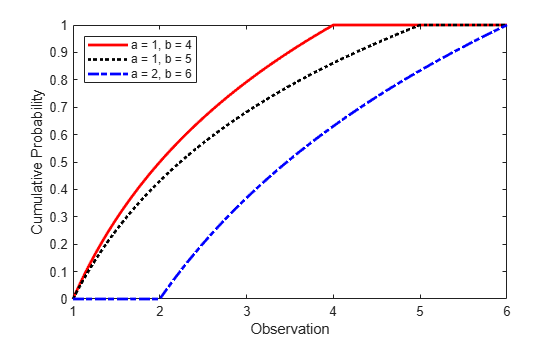## Loguniform Distribution

### Overview

The loguniform distribution (also called the reciprocal distribution) is a two-parameter distribution. This distribution has a probability density function that is proportional to the reciprocal of the variable value within its two bounding parameters (lower and upper limits of its support).

Create a probability distribution object `LoguniformDistribution` by specifying parameter values (`makedist`). Then, use object functions to evaluate the distribution, generate random numbers, and so on.

### Parameters

The loguniform distribution uses the following parameters.

ParameterDescriptionSupport
aLower limit0 < a < b
bUpper limit a < b <

### Probability Density Function

The probability density function (pdf) of the loguniform distribution is

`$f\left(x|a,b\right)=\left\{\begin{array}{c}\left(\frac{1}{x*\mathrm{log}\left(\frac{b}{a}\right)}\right)\text{ };\text{ }0`

For an example, see Compute and Plot Loguniform Distribution pdf.

### Cumulative Distribution Function

The cumulative distribution function (cdf) of the loguniform distribution is

`$F\left(x|a,b\right)=\left\{\text{ }\begin{array}{c}\text{ }\text{ }0\text{ }\text{ };\text{ }\text{ }x`

The result p is the probability that a single observation from a loguniform distribution with parameters a and b falls in the interval [a x].

For an example, see Compute Loguniform Distribution cdf.

### Descriptive Statistics

The mean of the loguniform distribution is $\mu =\frac{b-a}{\mathrm{log}\left(\frac{b}{a}\right)}$ .

The variance of the loguniform distribution is ${\sigma }^{2}=\frac{\mathrm{log}\left(\frac{b}{a}\right)\left({b}^{2}-{a}^{2}\right)-2{\left(b-a\right)}^{2}}{2{\left[\mathrm{log}\left(\frac{b}{a}\right)\right]}^{2}}$.

### Examples

#### Compute and Plot Loguniform Distribution pdf

Create three loguniform distribution objects with different parameters.

`pd1 = makedist('Loguniform') % Loguniform distribution with default parameters a = 1 and b = 4`
```pd1 = LoguniformDistribution Loguniform distribution Lower = 1 Upper = 4 ```
```pd2 = makedist('Loguniform','lower',1,'upper',5); % Loguniform distribution with a = 1 and b = 5 pd3 = makedist('Loguniform','lower',2,'upper',6); % Loguniform distribution with a = 2 and b = 6```

Compute the pdfs for the three loguniform distributions.

```x = 0:.01:6; pdf1 = pdf(pd1,x); pdf2 = pdf(pd2,x); pdf3 = pdf(pd3,x);```

Plot the pdfs on the same axis.

```figure; plot(x,pdf1,'r','LineWidth',2); hold on; plot(x,pdf2,'k:','LineWidth',2); plot(x,pdf3,'b-.','LineWidth',2); legend({'a = 1, b = 4','a = 1, b = 5','a = 2, b = 6'},'Location','northwest'); xlabel('Observation') ylabel('Probability Density') hold off;```The distribution density is proportional to the reciprocal of the variable value within a and b, hence, the pdf value decreases as the value of the variable increases.

#### Compute Loguniform Distribution cdf

Create three loguniform distribution objects with different parameters.

```pd1 = makedist('Loguniform'); % Loguniform distribution with default parameters a = 1 and b = 4 pd2 = makedist('Loguniform','lower',1,'upper',5); % Loguniform distribution with a = 1 and b = 5 pd3 = makedist('Loguniform','lower',2,'upper',6); % Loguniform distribution with a = 2 and b = 6```

Compute the cdfs for the three loguniform distributions.

```x = 1:.01:6; cdf1 = cdf(pd1,x); cdf2 = cdf(pd2,x); cdf3 = cdf(pd3,x);```

Plot the cdfs on the same axis.

```figure; plot(x,cdf1,'r','LineWidth',2); hold on; plot(x,cdf2,'k:','LineWidth',2); plot(x,cdf3,'b-.','LineWidth',2); legend({'a = 1, b = 4','a = 1, b = 5','a = 2, b = 6'},'Location','NW'); xlabel('Observation') ylabel('Cumulative Probability') hold off;```#### Transform Standard Uniform Sample to Loguniform Sample

Create a standard uniformly distributed sample of size 30.

` p = random('Uniform',0,1,30,1);`

Compute the loguniform sample with support (2,7) corresponding to the standard uniform values in `p`.

`logunifval = icdf('Loguniform',p,2,7);`

Alternatively, first create a loguniform distribution object with support (2,7) and then use it in the call to `icdf`.

`logunifpd = makedist('Loguniform', "Lower",2,"Upper",7)`
```logunifpd = LoguniformDistribution Loguniform distribution Lower = 2 Upper = 7 ```
`logunifval2 = icdf(logunifpd,p);`

#### Generate Random Numbers from Loguniform Distribution

To generate random numbers from a loguniform distribution, you must first create a loguniform distribution object. Create a loguniform distribution object with support (3,10).

`pd = makedist("Loguniform",3,10)`
```pd = LoguniformDistribution Loguniform distribution Lower = 3 Upper = 10 ```

Generate a 3-by-4 matrix of random numbers from the loguniform distribution.

`R = random(pd,3,4)`
```R = 3×4 8.0006 9.0096 4.1951 9.5861 8.9277 6.4235 5.7953 3.6269 3.4956 3.3738 9.5013 9.6521 ```

### Related Distributions

• Uniform Distribution — The continuous uniform distribution is a two-parameter distribution that has parameters a (lower limit) and b (upper limit). If X has a loguniform distribution within the support a and b, then log(X) has a uniform distribution between log(a) and log(b).